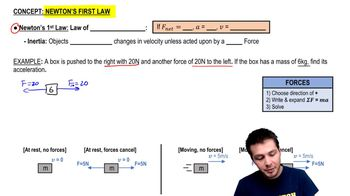Start typing, then use the up and down arrows to select an option from the list.
3:53 minutes
Problem 4a
Textbook Question

# A small 8.00-kg rocket burns fuel that exerts a time-varying upward force on the rocket (assume constant mass) as the rocket moves upward from the launch pad. This force obeys the equation F = A + Bt2. Measurements show that at t = 0, the force is 100.0 N, and at the end of the first 2.00 s, it is 150.0 N. (a) Find the constants A and B, including their SI unitsVerified Solution
This video solution was recommended by our tutors as helpful for the problem above.
298views